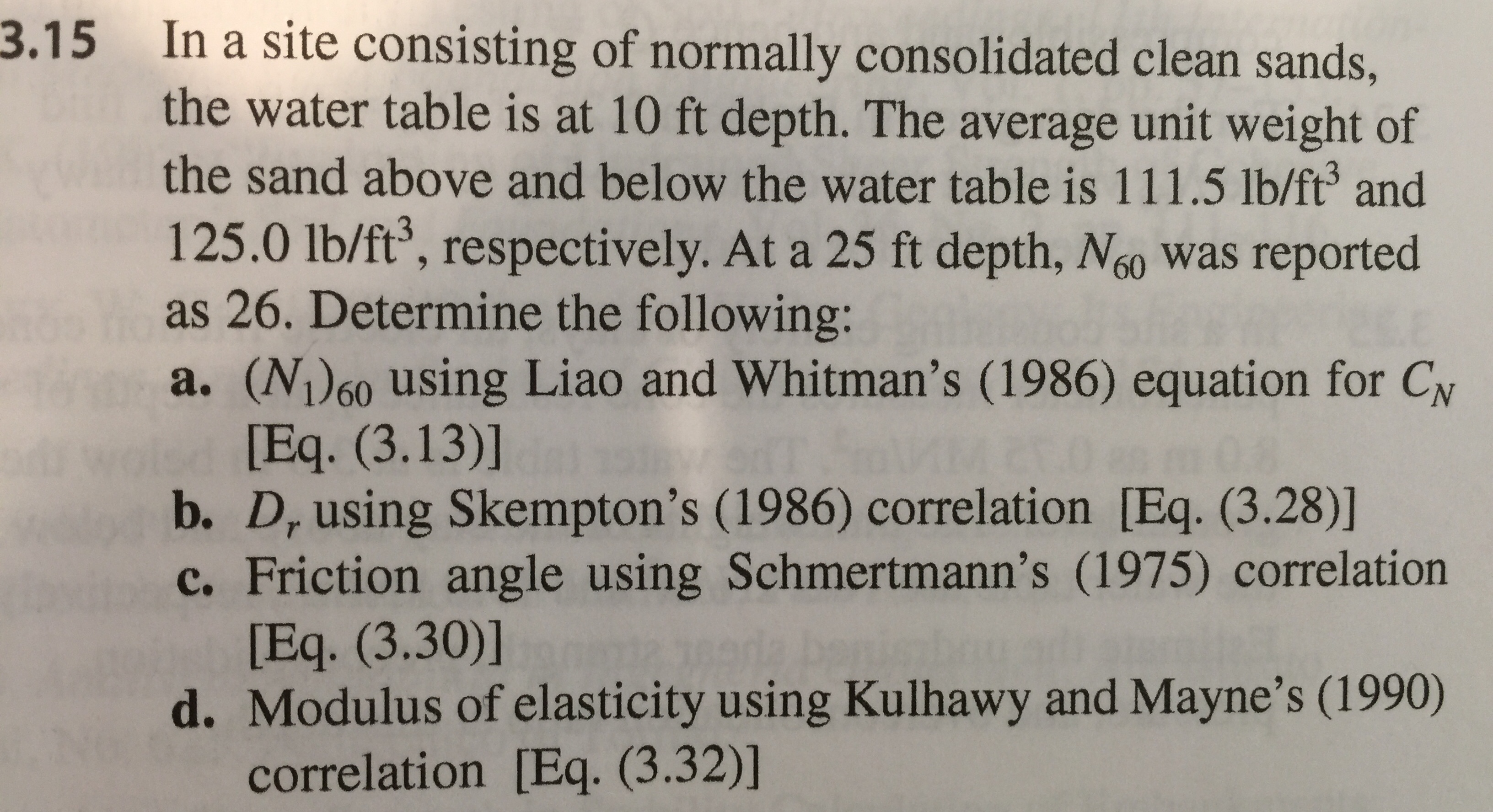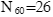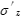3.15In a site consisting of normally consolidated clean sands,the water table is at 10 ft depth. The average unit weight ofthe sand above and below the water table is 111.5 lb/ft3 and125.0 lb/ft3, respectively. At a 25 ft depth, Nso was reportedas 26. Determine the following:a. (N160 using Liao and Whitman's (1986) equation for CN[Eq. (3.13)]b. D, using Skempton's (1986) correlation [Eq. (3.28)]c. Friction angle using Schmertmann's (1975) correlation[Eq. (3.30)]d. Modulus of elasticity using Kulhawy and Mayne's (1990)correlation [Eq. (3.32)]

Questionhelp_outlineImage Transcriptionclose3.15 In a site consisting of normally consolidated clean sands, the water table is at 10 ft depth. The average unit weight of the sand above and below the water table is 111.5 lb/ft3 and 125.0 lb/ft3, respectively. At a 25 ft depth, Nso was reported as 26. Determine the following: a. (N160 using Liao and Whitman's (1986) equation for CN [Eq. (3.13)] b. D, using Skempton's (1986) correlation [Eq. (3.28)] c. Friction angle using Schmertmann's (1975) correlation [Eq. (3.30)] d. Modulus of elasticity using Kulhawy and Mayne's (1990) correlation [Eq. (3.32)] fullscreen
Step 1

Given :Step 2

a. Using Liao and Whitman\\\'s (1986) equationis vertical effective stress where the sample was recovered.

Step 3

is the submerged unit weight of water

...

Want to see the full answer?

See Solution

Want to see this answer and more?

Our solutions are written by experts, many with advanced degrees, and available 24/7

See Solution
Tagged in

Civil Engineering